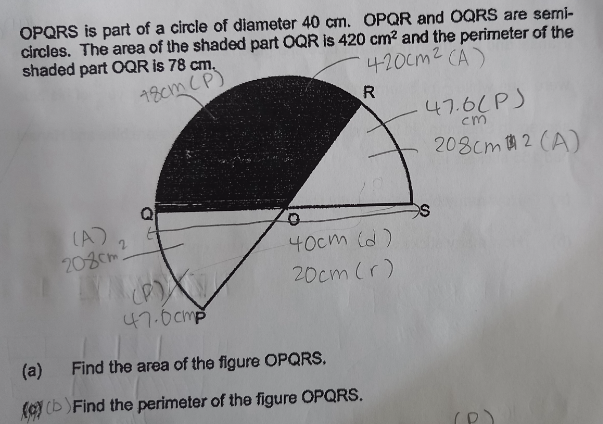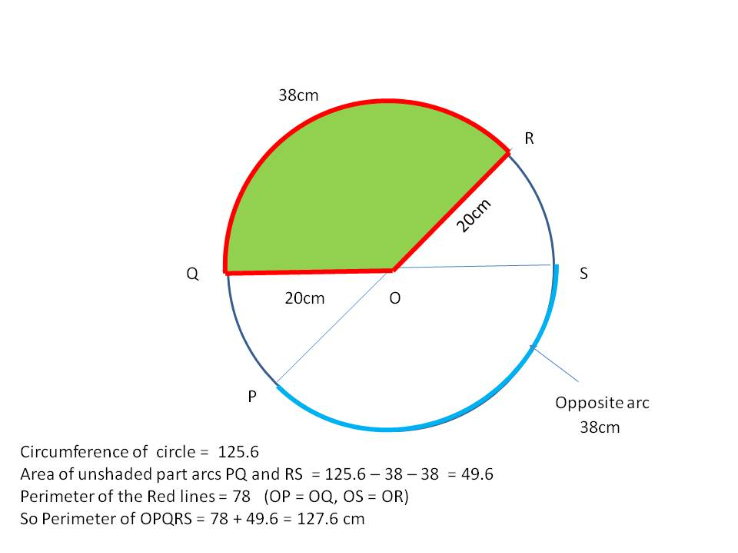# QuestionThank you!

Taking π =3.14

(a)  Area of circle = πr2  = 400π

Area of shaded part = 420

Area of the part opp the shaded part = 420 (vertically opposite)

So area of non-shaded part = 400π – 420 -420 = 400π – 840 = 1256 – 840 = 416

Hence area of OPQRS = 420 + 416 = 836cm2

(b) Circumference of circle = 2π r = 2 x 3.14 x 20 = 125.6

Arc of shaded part = 78-20-20 = 38   Opposite side arc = 38

Hence length of arc for unshaded part = 125.6 – 38 – 38 = 49.6

Therefore, perimeter of OPQRS = 78 + 49.6 = 127.6cm